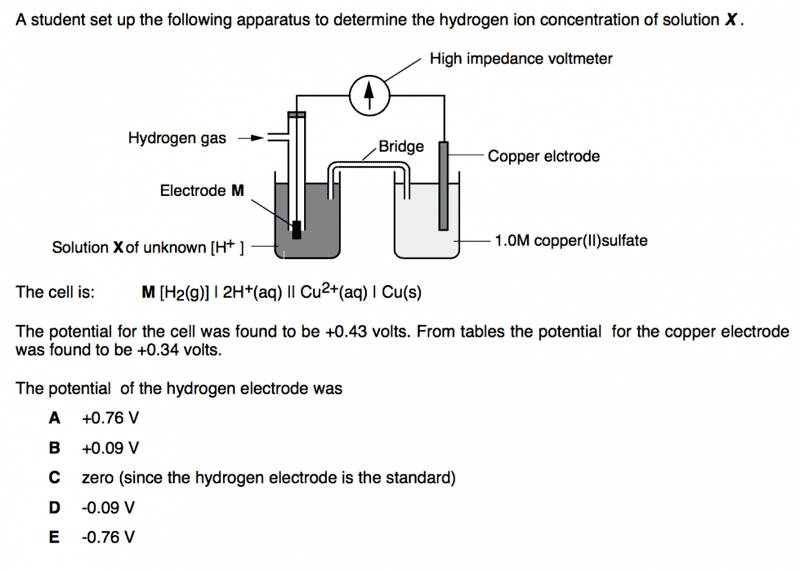# Potential of hydrogen electrode

## Homework Statement## The Attempt at a Solution

From the calculation of the potential of the cell and the potential for the copper electrode, it states that the hydrogen electrode has a potential of -0.09 V. But I thought hydrogen electrode is standard so it should be zero. Therefore I am not sure between C and D. Can someone explain which one is right and why?

Cheers!

Borek
Mentor
But I thought hydrogen electrode is standard so it should be zero.

What does it mean "standard electrode"? What does it mean "standard potential"?

Standard electrode is a standard hydrogen electrode. Standard potential is the difference in voltage between the standard hydrogen electrode and the concerning electrode. I guess the hydrogen electrode could be non standard as you don't know it's solution's molarity and what M is. This means the answer is D?

Cheers!

Borek
Mentor
Standard electrode is a standard hydrogen electrode. Standard potential is the difference in voltage between the standard hydrogen electrode and the concerning electrode.

"Standard is a standard" is a tautology and it doesn't add much to the problem.

What is the definition of the standard potential?

Actually it seems like you are aware of what is important here, but you failed to mention it correctly.

•TT0
What is the definition of the standard potential?
I am not too sure. I think standard potential is the reducing strengths of the other electrodes relative to the standard hydrogen electrode.

Borek
Mentor
I am not too sure.

•TT0
Standard potential is the measure of the energy per unit charge which is available from the oxidation/reduction reactions of each electrode relative to the standard hydrogen electrode. So as the hydrogen electrode is not the standard one, it's reduction potential can be non-zero so D is the answer?

Cheers!

Borek
Mentor
Sadly, this definition doesn't touch on a very important point - standard potential requires the system to be in the standard state.

•TT0
I see, thanks!